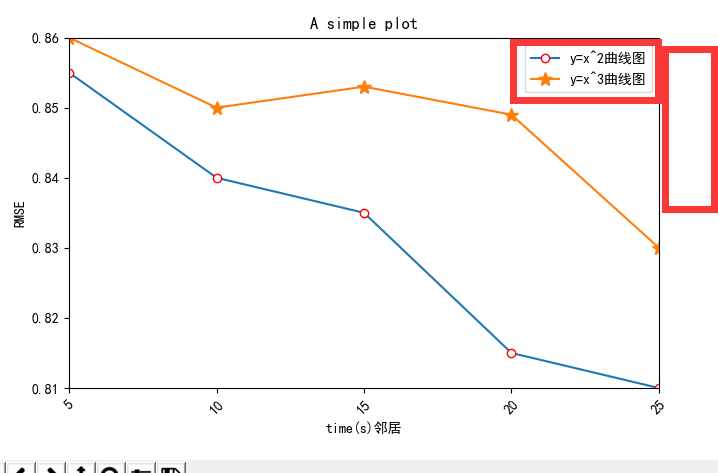python matplotlib.pyplot画折线图python画折线图，想要把每条线的标志部分移到外面的空白红色框中，该如何做

3个回答

plt.legend(bbox_to_anchor=(1.01,0.2), loc=3,ncol=1, mode="expand", borderaxespad=0.)python 使用matplotlib.pyplot 画折线图
【Python】使用matplotlib.pyplot实现画折线图的一个实用示例
Python实现画折线图的一个简单示例 # coding=utf-8 import numpy as np import matplotlib.pyplot as plt import matplotlib.font_manager as fm from scipy import interpolate font = 'TIMESBD.TTF' #字体文件，可使用自己电脑自带的其他字体文件 m...
python 画折线图
import matplotlib.pylab as pl def drew_lines(): x = [1,2,3,4,5,6,7,8,9,10,11,12,13] y = [0, 1, 0, 2, 4, 6, 8, 6, 8, 8, 9, 11, 12] pl.plot(x,y) pl.grid(True) pl.scatter(x, y) p...
python 画柱状图折线图

from numpy.random import randnimport matplotlib.pyplot as pltplt.style.use('ggplot')     #输入Y1值，定义X1的范围y1 = [0.8,0.4,0.2,0.1,0.05,0.025,0.0125,0.00625,0.0031,0.0016]x1 = range(0,10)fig = plt.figure()a...

#!/usr/bin/python3 # -*- coding: utf-8 -*- # __author__ = &quot;Mr.chen&quot; # Date: 2018/6/7 import json import matplotlib.pyplot as plt # 获取的监控数据，字典格式保存在文件中 new_dict = {} with open('json.txt', 'r', encodi...
python画折线图

python画图库之matplotlib.pyplot详细使用方法说明

Python 画股票收盘价折线图
# from __future__ import(absolute_import,division,print_function,unicode_literals) # try: # from urllib2 import urlopen #python 2.x # except ImportError: # from urllib.request import urlopen ...
python数据可视化之画折线图，散点图

[code=&quot;php&quot;] [/code] ShowChart.php [code=&quot;html&quot;] //异步请求 function Ajax(url) { AjaxObj = getXMLHTTPRequest(); AjaxObj.open(&quot;Get&quot;,url+&quot;?&amp;sid=&quot;+Math.random() ,true); ...
【python数据可视化笔记】——matplotlib.pyplot()

Python 画多个曲线的折线图（matplotlib.pyplot.plot）
这里我利用的是matplotlib.pyplot.plot的工具来绘制折线图，这里先给出一个段代码和结果图： # -*- coding: UTF-8 -*- import numpy as np import matplotlib as mpl import matplotlib.pyplot as plt #这里导入你自己的数据 #...... #...... #x_axix，tra...
python操作matplotlib画折线图显示数据

python画折线图坐标带时间

python导入csv文件画折线图
#导包import matplotlib.pyplot as pltimport pandas as pdimport timedata=pd.read_csv('filename')#导入csv文件y=data['number'].T.values#设置y轴数值 ,.T是转置x=[]array=[0,len(y)]for i in array:      x[i]=time.mktime(tim...

python 画折线图并通过邮件转发

python中 matplotlib.pyplot 库中 imshow 方法——画灰度图

python的str默认是ascii编码，和unicode编码冲突，因此经常会报以下错误：UnicodeDecodeError: ‘ascii’ codec can’t decode byte 0xe5 in position 108: ordinal not in range(128)解决这个问题很简单，只要在代码加入以下语句：import sys reload(sys) sys.setdefa...

teechart 和 Hight-Speed Charting 的测试学习
Android 画个折线图

D3.js画折线图

canvas 画折线图方法
function creatCanvas(){ //需要传入的数据 var data = [80,92,104,110,68,50,45]; //需要传入的x坐标 var time =["11","1.1","13.1","14.1","15.1","16.1","17.1"]; // 获取上下文 va
ggplot2 画折线图

AChartEngine画折线图
[img] [img]http://dl.iteye.com/upload/attachment/0063/8934/c730f30e-daa5-3973-b408-469bf6adb1fa.jpg[/img] [/img] 就一个Activity [code=&quot;java&quot;]package com.zzl.test; import java.util.ArrayList; im...

package com.app.map.widget; import android.content.Context; import android.graphics.Bitmap; import android.graphics.Canvas; import android.graphics.Color; import android.graphics.DashPathEffect; imp...
matplotlib画简单的折线图

jfreechart画折线图问题

[请教] VBA画折线图

Android画折线图和柱状图
Android画折线图和柱状图---源码，源码中附有详细解释，能正确运行，还有程序效果图。
c#画折线图

jfreechart画折线图

achartengine画折线图
achartengine画折线图。横坐标如何自动显示年月日。？？？？现在是将横坐标手工设置10个点。
OWC画折线图的问题

vb画折线图

Matplotlib画折线图
Matplotlib画折线图 有一些离散点，想看看这些点的变动趋势： import matplotlib.pyplot as plt x1 = [1, 2, 3, 4, 5, 6, 7, 8, 9, 10, 11, 12, 13] y1=[30,31,31,32,33,35,35,40,47,62,99,186,480] x2 = [1, 2, 3, 4, 5, 6, 7, 8, 9,
vba excel 画折线图
vbs:  Sub dttp() On Error Resume Next Dim rowend&amp;amp;, arr, i&amp;amp;, myShap As Shape, str As String, lastcol As Integer rowend = Range(&quot;a&quot; &amp;amp; Rows.Count).End(xlUp).Row ...
Canvas和Paint画折线图
Canvas和Paint实现简单的并且可扩展性十分强的折线图